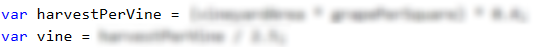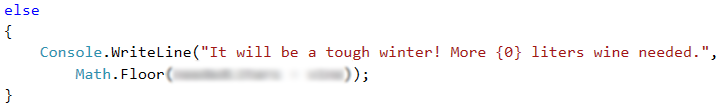# Problem: Harvest

In a vineyard with area X square meters, 40% of the harvest goes for wine production. Y kilograms of grapes are extracted from 1 $m^2$ vineyard. 2,5 kg of grapes are needed for 1 liter of wine. The wanted quantity of wine for sale is Z liters.

Write a program that calculates how much wine can be produced and whether that quantity is enough. If it is enough, the rest is divided between the vineyard workers equally.

## Input Data

The input data is read from the console and consists of exactly 4 lines:

• First line: X $m^2$ – the vineyard size – an integer in the range of [10 … 5000].
• Second line: Y grapes for one $m^2$ – an integer in the range of [0.00 … 10.00].
• Third line: Z needed liters of wine – an integer in the range of [10 … 600].
• Fourth line: number of workers – an integer in the range of [1 … 20].

## Output Data

The following has to be printed on the console:

• If the produced wine is less than the needed quantity:
• “It will be a tough winter! More {insufficient wine} liters wine needed.
* The result has to be rounded down to the nearest integer.
• If the produced wine is more than the needed quantity:
• “Good harvest this year! Total wine: {total wine} liters.”
* The result has to be rounded down to the nearest integer.
• “{Wine left} liters left -> {wine for one worker} liters per person.”
* Both of the results have to be rounded up to the higher integer.

## Sample Input and Output

Input Output Input Output
650
2
175
3
Good harvest this year! Total wine: 208 liters.
33 liters left -> 11 liters per person.
1020
1.5
425
4
It will be a tough winter! More 180 liters wine needed.

## Hints and Guidelines

In order to solve the problem, we will read the input data. Then, we will write a few conditional statements and do some calculations. Finally, we will print the result.

### Processing the Input Data

First, we have to check what the input data will be, so that we can choose what variables we will use.### Performing the Calculations

To solve the problem, based on the input data, we have to calculate how many liters of wine will be produced. From the task requirements, we see that in order to calculate the quantity of wine in liters, we firstly have to find the quantity of grapes in kilograms, which will be get from the harvest. For that, we will declare a variable that keeps a value, equal to 40% of the result from the multiplication of the vineyard area by the quantity of grapes, which is extracted from 1 $m^2$.

After having done these calculations, we are ready to calculate the quantity of wine in liters that will be produced from the harvest as well. For that, we declare one more variable that stores that quantity, which in order to calculate, we have to divide the quantity of grapes in kg by 2.5.### Checking the Conditions and Processing Output Data

After having done the necessary calculations, the next step is to check whether the liters of wine that have been produced, are enough. For that we will use a simple conditional statement of the if-else type and we will check whether the liters of wine from the harvest are more than or equal to the needed liters.

If the condition returns true, from the task requirement we see that on the first line we have to print the wine that has been produced from the harvest. That value has to be rounded down to the nearest integer, which we will do by using both the method Math.Floor(…) and a placeholder when printing it.

On the second line we have to print the results by rounding them up to the higher integer, which we will do by using the method Math.Ceiling(…). The values that we have to print are the quantity of wine left and the quantity that each worker gets. The wine left is equal to the difference between the produced liters of wine and the needed liters of wine. We calculate the value of that quantity in a new variable, which we declare and initialize in the if condition body, before printing the first line. We calculate the quantity of wine that each worker gets by dividing the wine left by the number of workers.If the condition returns false, we have to print the difference between the needed liters and the liters of wine produced from the harvest. There is a specification that the result has to be rounded down to the nearest integer, which we will do by using the method Math.Floor(…).